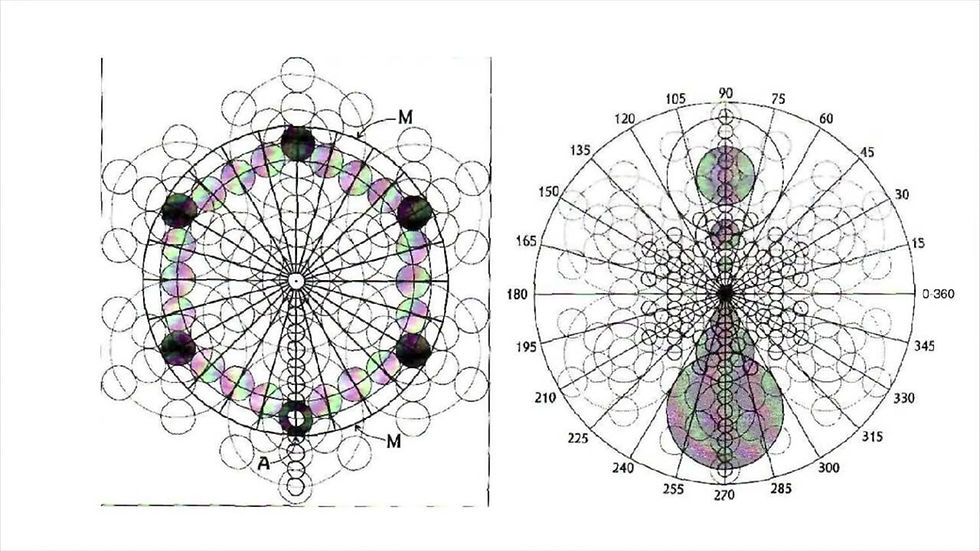top of page
Search

# Infinite tetrahedron grid part 2

The infinite dimensional E8 lie group is the infinite fractal 64 tetrahedron grid which is the infinite fractal fruit of life which is the cosmic tetractys which encodes 8 tree of life(E8xE8)The infinite fractal fruit of life(infinite cosmic tetractys) encodes all super strings and the 12 dimensions(vibrational dimensions):The infinite fractal 64 tetrahedron grid is the infinite tetrahedron grid which is made out of infinite infinite fractal dodecahedron star which is the 120-cell

Enneagram=(star tetrahedron)=(tree of life(E8xE8))=tetragrammaton=tetractys=120-cell

(Infinite tetrahedron grid)=(xen particle)

(64 tetrahedron grid)=E8